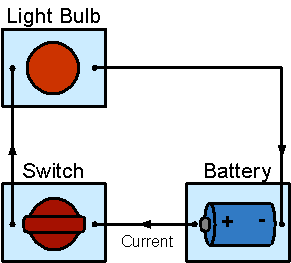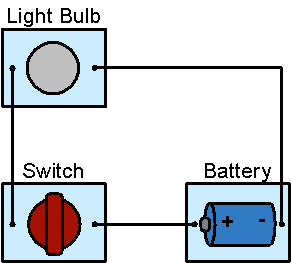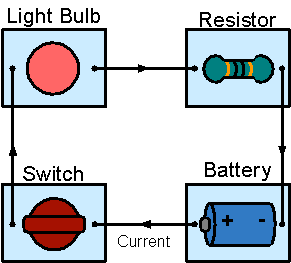# Direct Current Circuit

DC-Circuits > Direct Current Circuit

Direct Current (DC) is the constant flow of Electric Charge from high to low Potential. In the history of electrical science, conventional Current was defined as a flow of Positive Charge.

A Direct Current circuit is a circuit that Electric Current flows through in one direction. DC is commonly found in many low-voltage applications, especially where these are powered by Battery. Most electronic circuits require a DC power supply.

A Direct Electric Current flows only when the Electric Circuit is closed, but it stops completely when the circuit is open.

A Switch is a device for making or breaking an Electric Circuit. While the Switch is closed, Figure 1(a), the circuit is closed and the Light Bulb turns On; while the Switch is opened, Figure 1(b), the circuit is open and the Light Bulb turns Off.Figure 1(a): Switch is closed, the circuit is closed and the Light Bulb turns OnFigure 1(b): Switch is opened, the circuit is open and the Light Bulb turns Off

According to Ohm's Law: the Current I in a (ideal) Resistor (or other ohmic device) is proportional to the applied Voltage V and inversely proportional to the Resistance R.

Ohm's Law: I = V / R

In other words, for a fixed Resistance (R), the greater the Voltage (V) across a Resistor, the more the Current (I) flowing through it; for a fixed Voltage across a Resistor, the more the Resistance of the Resistor, the less the Current flowing through it.

In Figure 2, a Resistor is added to the Direct Current Circuit, the total Resistance (R) of the circuit becomes larger but the Power Supply Voltage (V) remains unchanged, therefore, the Current (I) flowing through the circuit is reduced. With less Current flowing through, the Light Bulb becomes dimmer.Figure 2: The Circuit contains a Battery, a Light Bulb, a Resistor and a Switch

DC-Circuits > Direct Current Circuit